# nth Root

The "nth Root" used n times in a multiplication gives the original value

## " nth ? "

1st, 2nd, 3rd, 4th, 5th, ... nth ...

Instead of talking about the "4th", "16th", etc, if we want to talk generally we say the "nth".

## The nth Root

 2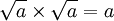Just like the square root is used two times in a multiplication to get the original value. 3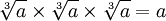And the cube root is used three times in a multiplication to get the original value. ... ... ... nThe nth root is used n times in a multiplication to get the original value.

So it is the general way of talking about roots
(so it could be 2nd, or 9th, or 324th, or whatever)

## The nth Root Symbol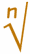This is the special symbol that means "nth root", it is the "radical" symbol (used for square roots) with a little n to mean nth root.

## Using it

We could use the nth root in a question like this:

Question: What is "n" in this equation?Answer: I just happen to know that 625 = 54, so the 4th root of 625 must be 5: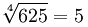Or we could use "n" because we want to say general things:

Example: When n is odd(we talk about this later).

## Why "Root" ... ?When you see "root" think "I know the tree, but what is the root that produced it?" Example: in √9 = 3 the "tree" is 9, and the root is 3.

## Properties

Now we know what an nth root is, let us look at some properties:

### Multiplication and Division

We can "pull apart" multiplications under the root sign like this: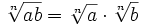(If n is even, a and b must both be ≥ 0)

This can help us simplify equations in algebra, and also make some calculations easier:

Example: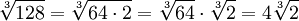It also works for division: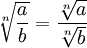(a≥0 and b>0)
(b cannot be zero, as we can't divide by zero)

Example:But we cannot do that kind of thing for additions or subtractions !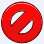Example: Pythagoras' Theorem saysa2 + b2 = c2

So we can calculate c like this:

c = √(a2 + b2)

Which is not the same as c = a + b, right?

It is an easy trap to fall into, so beware. It also means that, unfortunately, additions and subtractions can be hard to deal with when under a root sign.

### Exponents vs Roots

An exponent on one side of the "=" can be turned into a root on the other side of the "=":If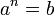then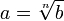(when n is even b must be ≥ 0)

Example: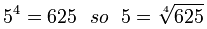### nth Root of a-to-the-nth-Power

When a value has an exponent of n and we take the nth root we get the value back again ...

 ... when a is positive (or zero):(when a ≥ 0)

Example:... or when the exponent is odd:(when n is odd)

Example:... but when a is negative and the exponent is even we get this:Did you see that −3 became +3 ?

 ... so we have:(when a < 0 and n is even)

(Note: |a| means the absolute value of a, in other words any negative becomes a positive)

Example:So that is something to be careful of! Read more at Exponents of Negative Numbers.

Here it is in a little table:

n is odd n is even
a ≥ 0a < 0### nth Root of a-to-the-mth-Power

Now let's see what happens when the exponent and root are different values (m and n).Example: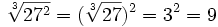So ... we can move the exponent "out from under" the nth root, which may sometimes be helpful.

But there is an even more powerful method ... we can combine the exponent and root to make a new exponent, like this: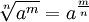Example: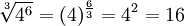That is because the nth root is the same as an exponent of (1/n):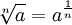Example: 2½ = √2 (the square root of 2)

You might like to read about Fractional Exponents next to find out why!